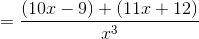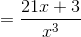## Example Questions

### Example Question #1 : How To Add Rational Expressions With A Common Denominator

Simplify the following rational expression: (9x - 2)/(x2) MINUS (6x - 8)/(x2)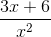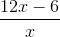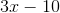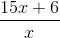Explanation:

Since both expressions have a common denominator, x2, we can just recopy the denominator and focus on the numerators. We get (9x - 2) - (6x - 8). We must distribute the negative sign over the 6x - 8 expression which gives us 9x - 2 - 6x + 8 ( -2 minus a -8 gives a +6 since a negative and negative make a positive). The numerator is therefore 3x + 6.

### Example Question #2 : How To Add Rational Expressions With A Common Denominator

Simplify the following rational expression: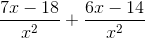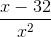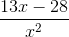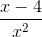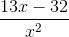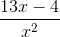Explanation:

Since both fractions in the expression have a common denominator of, we can combine like terms into a single numerator over the denominator: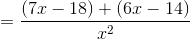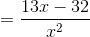### Example Question #3 : How To Add Rational Expressions With A Common Denominator

Simplify the following rational expression: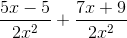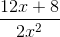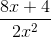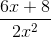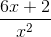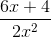Explanation:

Since both rational terms in the expression have the common denominator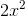, combine the numerators and simplify like terms: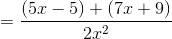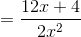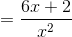### Example Question #4 : How To Add Rational Expressions With A Common Denominator

Simplify the following expression: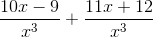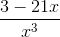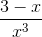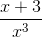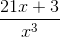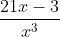Explanation:

Since both terms in the expression have the common denominator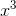, combine the fractions and simplify the numerators: